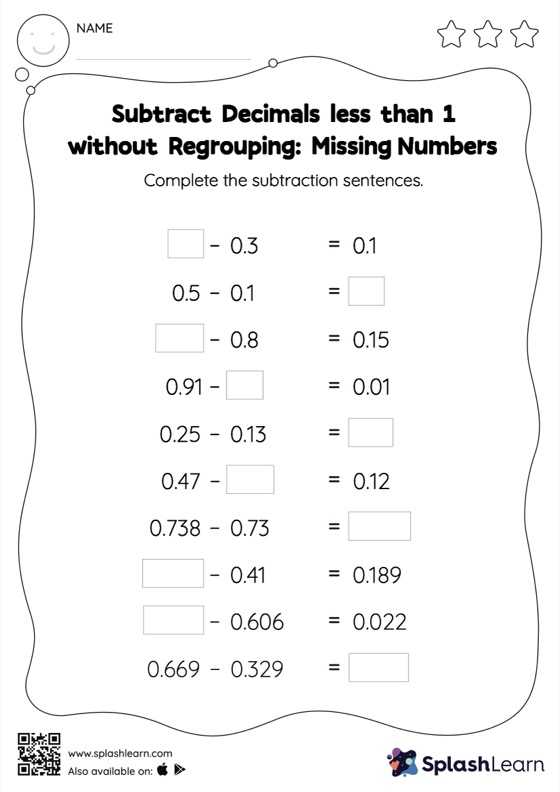# Subtract Decimals less than 1 without Regrouping: Missing Numbers Worksheet

Home > Subtract Decimals less than 1 without Regrouping: Missing NumbersThis subtract decimals less than 1 without regrouping worksheet consists of tasks to help your young mathematician develop fluency with subtraction. While subtracting decimals, students use the relationship between addition and subtraction to find the missing number. They do not need to regroup numbers in subtract decimals less than 1 without regrouping worksheet.